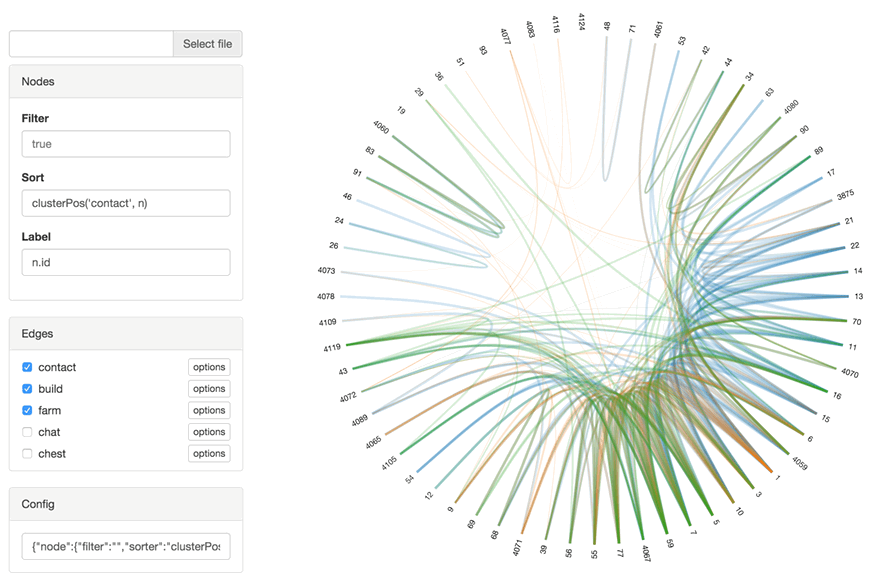The graph miner tool enables interactive exploration of player relationships. Several weighted graphs can be visualized at once. The players, represented the vertices of the graph, can be filtered and sorted using JavaScript expressions. The relationship graphs connect players pairwise. Edges can be filtered and their weight can be set by custom JavaScript expressions also.Try the interactive demo or download the source code.

# Manual

Works best with Chrome. Use Enter to update the Graph after changing options.

## Graph Definition

The graph is defined in `data/graph.json.js`. The nodes are defined as `graph.node.id = {id: id}`. Additional properties can be defined at will. Edge groups are defined as `graph.edge.groupName = g`. They are represented as sparse weighted adjacency matrix: `g[idSrc][idDst] = weight`.

## Player Node Properties

The following properties are available for player nodes

• n.id node id
• n.uid Mojang account uuid [String]
• n.name player name [String]
• n.tLastEvent time last recorded event [epoch]
• n.tActive active gameplay [seconds]
• n.tSocial active near other players [seconds*nPlayers]
• n.nBlockBreak number of blocks broken [blocks]
• n.nBlockPlace number of blocks placed [blocks]
• n.sMove distance moved [blocks]

## Expressions

The fields Filter, Sort, Label and Width accept JavaScript expressions. Depending on context, a node object `n` or a value `v` can be accessed.

In addition to standard JavaScript functions, some helper functions are available:

• edgeSum(Node n) returns the sums of the weights from all outgoing edges. Can be used for node sorting and filtering.
• uScale(v) returns `1-(1/(v+1))` which can be used to normalize edge widths.
• clusterPos(String edgeGroup, Node n) returns a node's position after sorting a hierarchical cluster consisting of the edges from edgeGroup. Can be used for node sorting.

## Node Options

### Filter

Expression to decide if a node is displayed (true) or not (false). The current node object can be accessed over the variable `n`.

Example To show all players active for more than one hour, enter `n.tActive > 60*60`.

### Sort

JavaScript expression returning either a string or a number which is used to sort the nodes. The current node object can be accessed over the variable `n`.

Example To sort the nodes by name, enter `n.name`.

### Label

JavaScript expression returning the content for the node label. The current node object can be accessed over the variable `n`.

Example To show player names, enter `n.name`.

## Edge Options

Edge groups can be hidden using the checkboxes in the edge group list. To access a edge group options, use the "options" button. Push it again to hide the options.

### Filter

Expression to decide if a edge is displayed (true) or not (false). The weight of the current edge can be accessed over the variable `v`.

Example To only show edges with weights over 9000, enter `v > 9000`.

### Width

Expression returning the with of the line representing the edge. The weight of the current edge can be accessed over the variable `v`.

Example To use a logarithmic scale, enter `Math.log(v/100)*2`.

### Helpers

• sumFilter puts `edgeSum('edgeGroup', n) != 0` into the node filter field, resulting in hiding all nodes with no outgoing edges.
• sumFilter puts `-edgeSum('edgeGroup', n)` into the node sort field, sorting the nodes by the sum of their outgoing edges.
• clusterSort puts `clusterPos('edgeGroup', n)` into the node sort field, sorting the nodes by hierarchical clustering.

## Config

JSON string containing the current options. Can be copy/pasted and saved elsewhere.